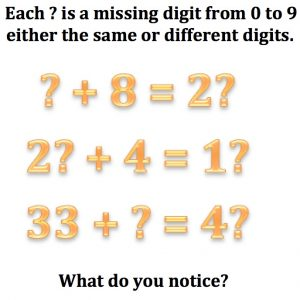You are a detective and you have to discover what digits the question marks stand for. They can be any digit from 0 to 9 and they can be the same digit or different digits.

How will you find the missing digits?

What do you notice?

How many solutions are there to the calculations?

Explain why this happens?

Now find the missing digits in these calculations:

(a) 3?6 + 5? = 383               (b) 8?3 – 7? = 815

What clues did you use? Explain how you solved the puzzles.

In the next two puzzles each of the digits must be different.

(c) Find the largest possible total for ?? + ?? =

Explain why it is the largest.

Find the smallest possible total and explain?

(d) What about ?? × ??

(e) Now find the missing digit in this division: 816 ÷ ? = 272